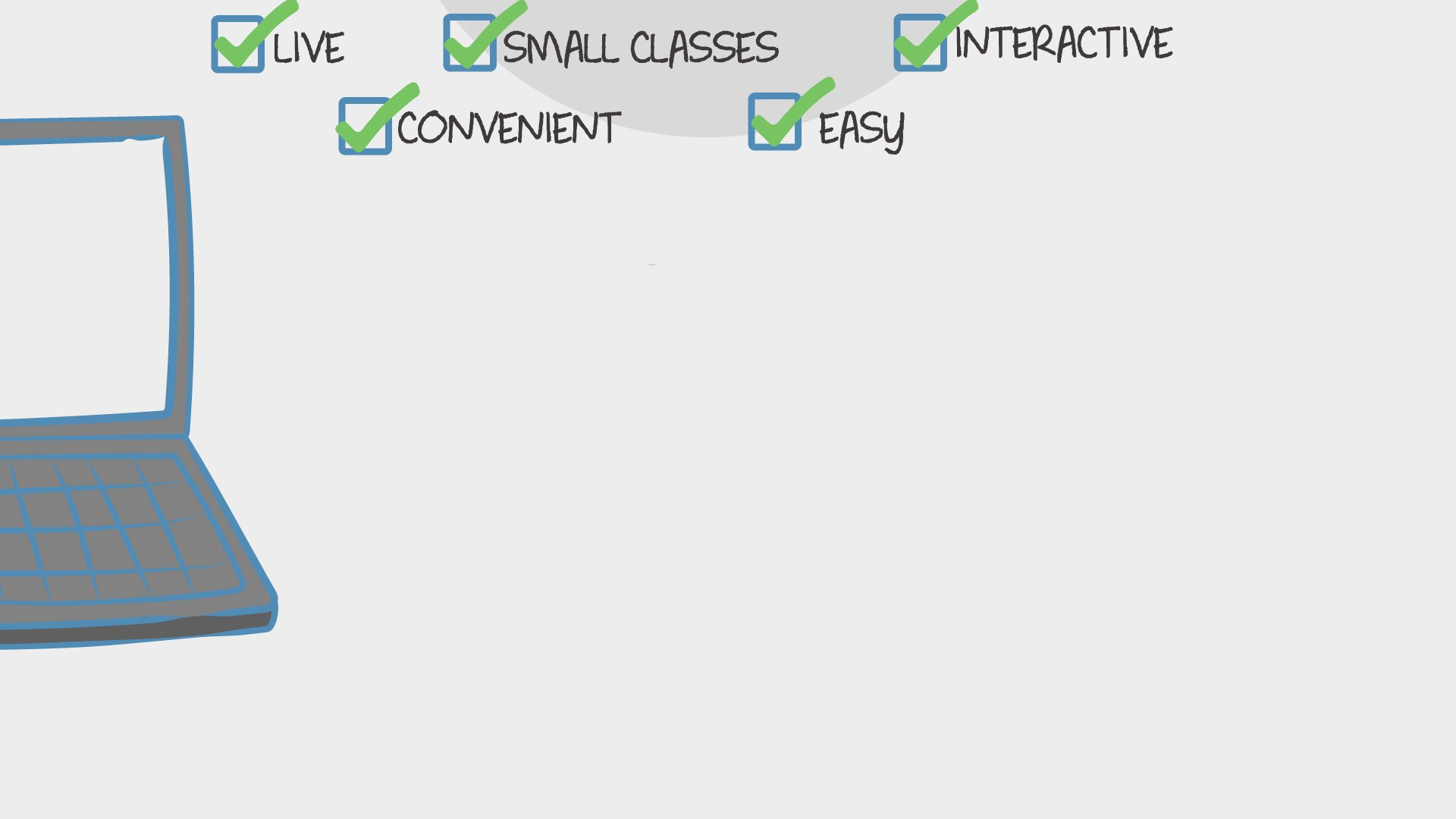Find Classes
Teach

Math

# Summer Step Up to Second Grade Math! (Second Grade Review or Preview)

In this On-going course we will look into second grade math topics that students need to review or a preview of for next year.Ms. Jamie
57 total reviews for this teacher
Completed by 6 learners
There are no upcoming classes.30 minutes
per class
Twice per week
every week
6-9
year olds
3-9
learners per class
per learner - per class

### How does an “Ongoing” course work?

Meets on a weekly schedule, join any week, no need to catch up on previous material
Live video chats, recorded and monitored for safety and quality
Discussions via classroom forum and private messages with the teacher
Automatic payment every Sunday, cancel any time
Great for clubs and for practicing skills### How Outschool Works### There are no open spots for this class.

You can request another time or scroll down to find more classes like this.

## Description

### Class Experience

```Students will be exposed to the following Common Core Standards.

Represent and solve problems involving addition and subtraction.
Operations and Algebraic Thinking
CCSS.MATH.CONTENT.2.OA.A.1
Use addition and subtraction within 100 to solve one- and two-step word problems involving situations of adding to, taking from, putting together, taking apart, and comparing, with unknowns in all positions, e.g., by using drawings and equations with a symbol for the unknown number to represent the problem.1
CCSS.MATH.CONTENT.2.OA.B.2
Fluently add and subtract within 20 using mental strategies.2 By end of Grade 2, know from memory all sums of two one-digit numbers.
Work with equal groups of objects to gain foundations for multiplication.
CCSS.MATH.CONTENT.2.OA.C.3
Determine whether a group of objects (up to 20) has an odd or even number of members, e.g., by pairing objects or counting them by 2s; write an equation to express an even number as a sum of two equal addends.
CCSS.MATH.CONTENT.2.OA.C.4
Use addition to find the total number of objects arranged in rectangular arrays with up to 5 rows and up to 5 columns; write an equation to express the total as a sum of equal addends.
Number Base Ten
CCSS.MATH.CONTENT.2.NBT.A.1
Understand that the three digits of a three-digit number represent amounts of hundreds, tens, and ones; e.g., 706 equals 7 hundreds, 0 tens, and 6 ones. Understand the following as special cases:CCSS.MATH.CONTENT.2.NBT.A.2
Count within 1000; skip-count by 5s, 10s, and 100s.
CCSS.MATH.CONTENT.2.NBT.A.3
Read and write numbers to 1000 using base-ten numerals, number names, and expanded form.
CCSS.MATH.CONTENT.2.NBT.A.4
Compare two three-digit numbers based on meanings of the hundreds, tens, and ones digits, using >, =, and < symbols to record the results of comparisons.
Use place value understanding and properties of operations to add and subtract.
CCSS.MATH.CONTENT.2.NBT.B.5
Fluently add and subtract within 100 using strategies based on place value, properties of operations, and/or the relationship between addition and subtraction.
CCSS.MATH.CONTENT.2.NBT.B.6
Add up to four two-digit numbers using strategies based on place value and properties of operations.
CCSS.MATH.CONTENT.2.NBT.B.7
Add and subtract within 1000, using concrete models or drawings and strategies based on place value, properties of operations, and/or the relationship between addition and subtraction; relate the strategy to a written method. Understand that in adding or subtracting three-digit numbers, one adds or subtracts hundreds and hundreds, tens and tens, ones and ones; and sometimes it is necessary to compose or decompose tens or hundreds.
CCSS.MATH.CONTENT.2.NBT.B.9
Explain why addition and subtraction strategies work, using place value and the properties of operations.
Geometry
Reason with shapes and their attributes.
CCSS.MATH.CONTENT.2.G.A.1
Recognize and draw shapes having specified attributes, such as a given number of angles or a given number of equal faces.1 Identify triangles, quadrilaterals, pentagons, hexagons, and cubes.
CCSS.MATH.CONTENT.2.G.A.2
Partition a rectangle into rows and columns of same-size squares and count to find the total number of them.
CCSS.MATH.CONTENT.2.G.A.3
Partition circles and rectangles into two, three, or four equal shares, describe the shares using the words halves, thirds, half of, a third of, etc., and describe the whole as two halves, three thirds, four fourths. Recognize that equal shares of identical wholes need not have the same shape.
Measurement and Data
Work with time and money.
CCSS.MATH.CONTENT.2.MD.C.7
Tell and write time from analog and digital clocks to the nearest five minutes, using a.m. and p.m.
CCSS.MATH.CONTENT.2.MD.C.8
Solve word problems involving dollar bills, quarters, dimes, nickels, and pennies, using \$ and ¢ symbols appropriately. Example: If you have 2 dimes and 3 pennies, how many cents do you have?
Represent and interpret data.
CCSS.MATH.CONTENT.2.MD.D.9
Generate measurement data by measuring lengths of several objects to the nearest whole unit, or by making repeated measurements of the same object. Show the measurements by making a line plot, where the horizontal scale is marked off in whole-number units.
CCSS.MATH.CONTENT.2.MD.D.10
Draw a picture graph and a bar graph (with single-unit scale) to represent a data set with up to four categories. Solve simple put-together, take-apart, and compare problems1 using information presented in a bar graph.
```
`Please contact me if you would like assigned homework. `
`1 hour per week in class, and maybe some time outside of class.`

## Teacher

Ms. Jamie
🇺🇸
Lives in the United States
World Traveler, Experienced Certified Teacher, Reading Coach, M. Ed.
57 total reviews
93 completed classes```Hello!